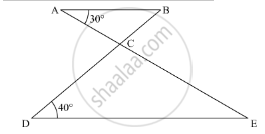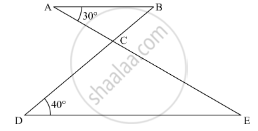Advertisement Remove all ads

# In the Given Figure, Ab || De. Find ∠Acd. - Mathematics

Answer in Brief

In the given figure, AB || DE. Find ∠ACD.Advertisement Remove all ads

#### Solution

In the given problem, AB || DE

We need to find ∠ACDNow, AB || DE and AEis the transversal, so using the property, “alternate interior angles are equal”, we get,

∠BAE = ∠AED

∠AED = 30

Further, applying angle sum property of the triangle

In ΔDCE

∠DCE  + ∠D + ∠E = 180

∠DCE + 40 + 30 = 180

∠DCE + 70 = 180

∠DCE = 180° - 70°

∠DCE = 110°

Further, ACE is a straight line, so using the property, “the angles forming a linear pair are supplementary”, we get,

∠ACD + ∠DCE = 180°

∠ACD + 110 = 180°

∠ACD = 180 - 110°

∠ACD = 70°

Therefore, ∠ACD = 70° .

Is there an error in this question or solution?
Advertisement Remove all ads

#### APPEARS IN

RD Sharma Mathematics for Class 9
Chapter 11 Triangle and its Angles
Exercise 11.2 | Q 5 | Page 21
Advertisement Remove all ads
Advertisement Remove all ads
Share
Notifications

View all notifications

Forgot password?
Course